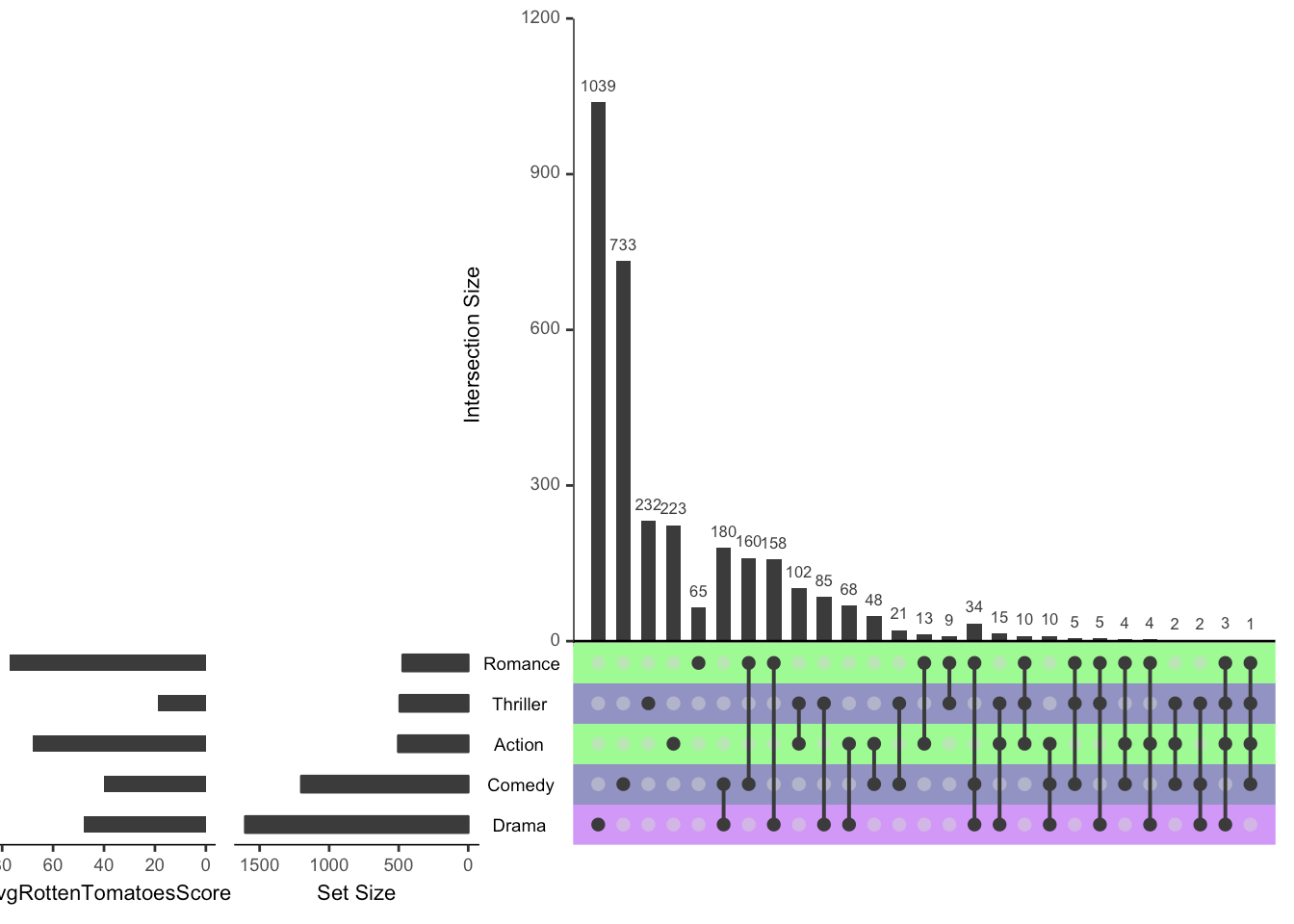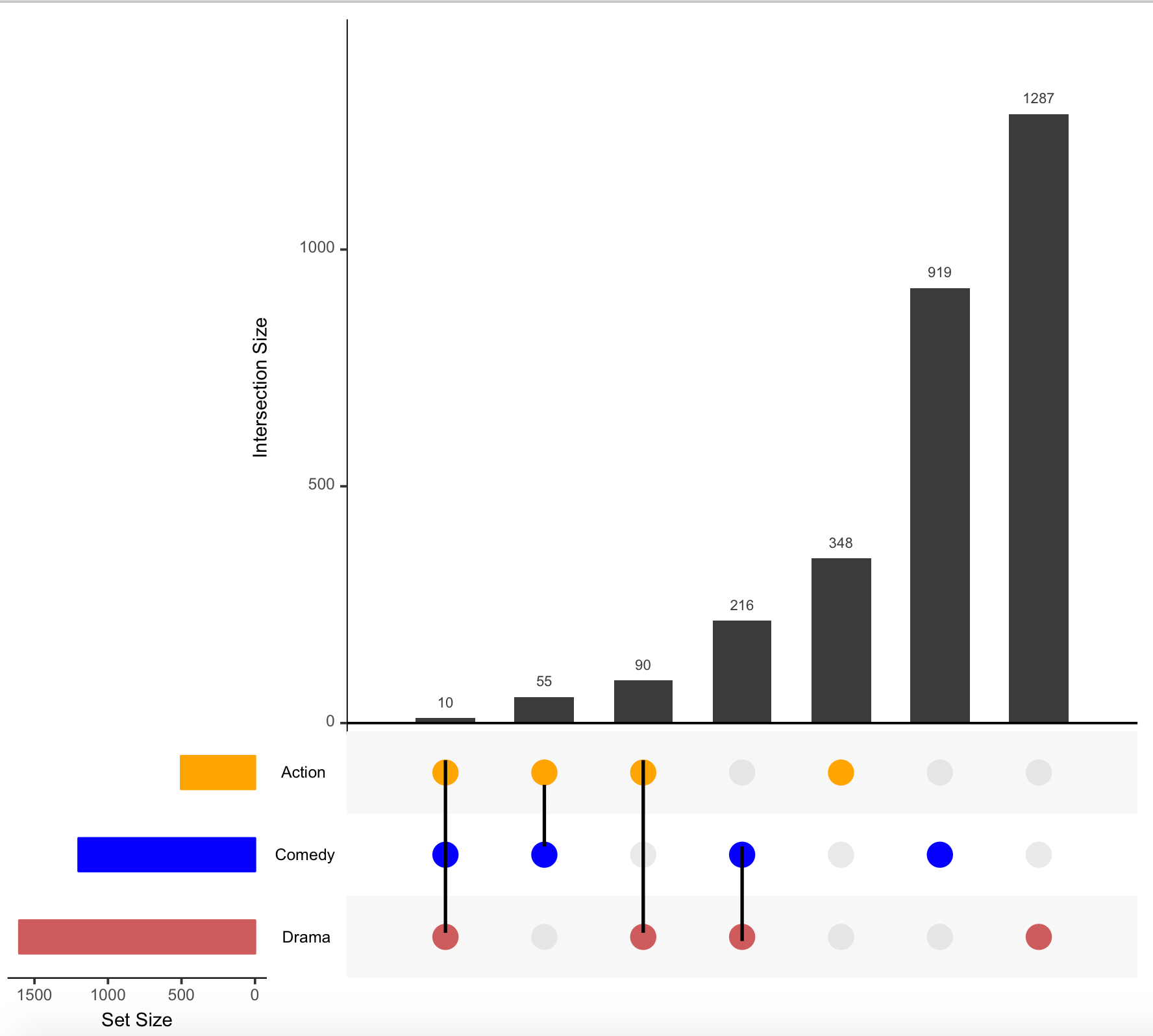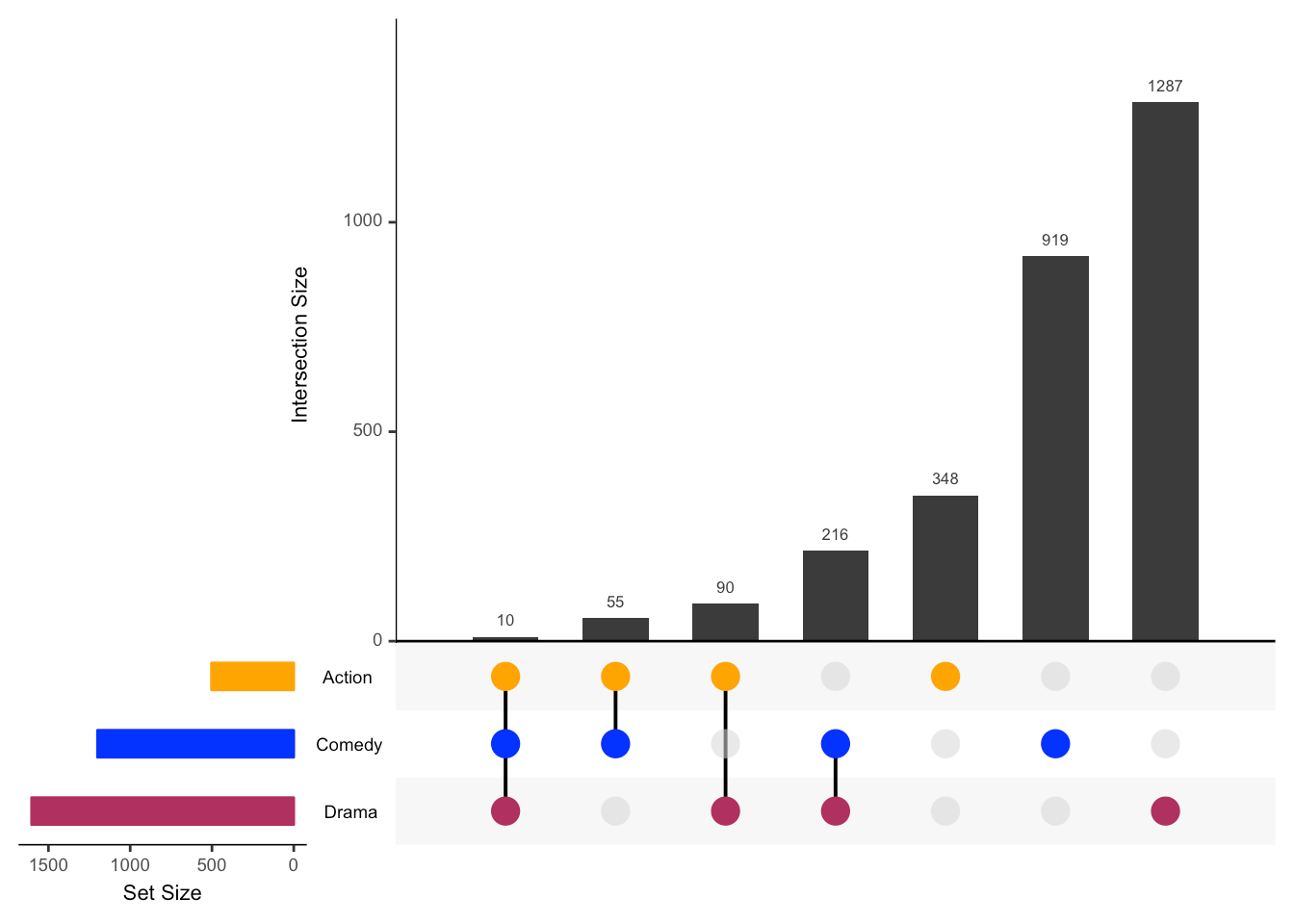# Hacking our way through UpSetR

Want to share your content on R-bloggers? click here if you have a blog, or here if you don't.

For our club meeting today we were going to summarize the Demystifying Data Science conference but we forgot that the videos are not released yet.

So we adjusted plans and decided to continue our work on the UpSetR (Gehlenborg, 2016) package by Nils Gehlenborg.

### What you can currently do

First, let’s install the version we used for this post:

``devtools::install_github('hms-dbmi/[email protected]')``

Our ultimate goal is to submit a pull request that enables `UpSetR` users to specify a color by row for the dots instead of the actual rows. We had already identified an example that we could work with.

``````library('UpSetR')
movies <- read.csv( system.file("extdata", "movies.csv", package = "UpSetR"),

require(ggplot2); require(plyr); require(gridExtra); require(grid);``````
``## Loading required package: ggplot2``
``## Loading required package: plyr``
``## Loading required package: gridExtra``
``## Loading required package: grid``
``````upset(movies,
sets = c("Action", "Comedy", "Drama"),
order.by="degree", matrix.color="blue", point.size=5,
sets.bar.color=c("maroon","blue","orange"))``````We also explored the set metadata vignette that includes examples such as the following one.

``````set.seed(20180727)

## Create the metadata object first
sets <- names(movies[3:19])
avgRottenTomatoesScore <- round(runif(17, min = 0, max = 90))
Cities <- sample(c("Boston", "NYC", "LA"), 17, replace = T)
"Romance")), ]``````
``````##        sets avgRottenTomatoesScore Cities
## 1    Action                     68 Boston
## 4    Comedy                     40    NYC
## 7     Drama                     48     LA
## 13  Romance                     77 Boston
## 15 Thriller                     19    NYC``````
``````accepted <- round(runif(17, min = 0, max = 1))
"Romance")), ]``````
``````##        sets avgRottenTomatoesScore Cities accepted
## 1    Action                     68 Boston        0
## 4    Comedy                     40    NYC        1
## 7     Drama                     48     LA        0
## 13  Romance                     77 Boston        1
## 15 Thriller                     19    NYC        0``````
``````## Now make the plot
column = "avgRottenTomatoesScore", assign = 20), list(type = "matrix_rows",
column = "Cities", colors = c(Boston = "green", NYC = "navy", LA = "purple"),
alpha = 0.5))))``````### Hacking our way

Using the `metadata` looked complicated to us and hopefully not necessary for what we are trying to accomplish. That is, we really wanted to change the colors of the circles in each row, not the rows themselves. So we found the GitHub repo with the code, plugged a laptop to a TV and started exploring as a group. We went the rabbit hole to see how the `matrix.color` argument got used. To actually hack our way through, we downloaded the latest version of the code using `git`.

``````git clone [email protected]:hms-dbmi/UpSetR.git
cd UpSetR``````

Next, we created the objects that match the default arguments of `upset()` by finding and replacing commas by semi-colons. Well, not all of the commas. Also, for inputs that specified a vector (mostly 2 options), we chose the first one to match the default R behavior. This way we could execute them and have them in our session.

``````## Default upset() arguments
nsets = 5; nintersects = 40; sets = NULL; keep.order = F; set.metadata = NULL; intersections = NULL;
matrix.color = "gray23"; main.bar.color = "gray23"; mainbar.y.label = "Intersection Size"; mainbar.y.max = NULL;
sets.bar.color = "gray23"; sets.x.label = "Set Size"; point.size = 2.2; line.size = 0.7;
mb.ratio = c(0.70,0.30); expression = NULL; att.pos = NULL; att.color = main.bar.color; order.by = 'freq';
decreasing = T; show.numbers = "yes"; number.angles = 0; group.by = "degree";cutoff = NULL;
queries = NULL; query.legend = "none"; shade.color = "gray88"; shade.alpha = 0.25; matrix.dot.alpha =0.5;
empty.intersections = NULL; color.pal = 1; boxplot.summary = NULL; attribute.plots = NULL; scale.intersections = "identity";
scale.sets = "identity"; text.scale = 1; set_size.angles = 0 ; set_size.show = FALSE ``````

Next, we did the same (commas to semicolons) for the inputs of the first example.

``````## Initial inputs on the first example
movies <- read.csv( system.file("extdata", "movies.csv", package = "UpSetR"),

## comma -> semicolon
data = movies; sets = c("Action", "Comedy", "Drama");
order.by="degree"; matrix.color="blue"; point.size=5;
sets.bar.color=c("maroon","blue","orange")``````

Now we were ready to start modifying some of the internal UpSetR (Gehlenborg, 2016) code.

### Hacking internals

The function `upset()` is pretty long and uses many un-exported functions from the package itself. In order to test thing quickly we added `UpSetR:::` calls before the un-exported functions. Here’s our modified version where we added a piece of code to modify the `Matrix_layout` object and add some colors.

``````## Piece of code we introduced
for(i in 1:3) {
j <- which(Matrix_layout\$y == i & Matrix_layout\$value == 1)
if(length(j) > 0) Matrix_layout\$color[j] <- c("maroon","blue","orange")[i]
}``````

Ok, here’s the full modified `upset()` function.

``````## Modified internal upset() code

startend <- UpSetR:::FindStartEnd(data)
first.col <- startend
last.col <- startend

if(color.pal == 1){
palette <- c("#1F77B4", "#FF7F0E", "#2CA02C", "#D62728", "#9467BD", "#8C564B", "#E377C2",
"#7F7F7F", "#BCBD22", "#17BECF")
} else{
palette <- c("#E69F00", "#56B4E9", "#009E73", "#F0E442", "#0072B2", "#D55E00",
"#CC79A7")
}

if(is.null(intersections) == F){
Set_names <- unique((unlist(intersections)))
Sets_to_remove <- UpSetR:::Remove(data, first.col, last.col, Set_names)
New_data <- UpSetR:::Wanted(data, Sets_to_remove)
Num_of_set <- UpSetR:::Number_of_sets(Set_names)
if(keep.order == F){
Set_names <- UpSetR:::order_sets(New_data, Set_names)
}
All_Freqs <- UpSetR:::specific_intersections(data, first.col, last.col, intersections, order.by, group.by, decreasing,
cutoff, main.bar.color, Set_names)
} else if(is.null(intersections) == T){
Set_names <- sets
if(is.null(Set_names) == T || length(Set_names) == 0 ){
Set_names <- UpSetR:::FindMostFreq(data, first.col, last.col, nsets)
}
Sets_to_remove <- UpSetR:::Remove(data, first.col, last.col, Set_names)
New_data <- UpSetR:::Wanted(data, Sets_to_remove)
Num_of_set <- UpSetR:::Number_of_sets(Set_names)
if(keep.order == F){
Set_names <- UpSetR:::order_sets(New_data, Set_names)
}
All_Freqs <- UpSetR:::Counter(New_data, Num_of_set, first.col, Set_names, nintersects, main.bar.color,
order.by, group.by, cutoff, empty.intersections, decreasing)
}
Matrix_setup <- UpSetR:::Create_matrix(All_Freqs)
labels <- UpSetR:::Make_labels(Matrix_setup)
#Chose NA to represent NULL case as result of NA being inserted when at least one contained both x and y
#i.e. if one custom plot had both x and y, and others had only x, the y's for the other plots were NA
#if I decided to make the NULL case (all x and no y, or vice versa), there would have been alot more if/else statements
#NA can be indexed so that we still get the non NA y aesthetics on correct plot. NULL cant be indexed.
att.x <- c(); att.y <- c();
if(is.null(attribute.plots) == F){
for(i in seq_along(attribute.plots\$plots)){
if(length(attribute.plots\$plots[[i]]\$x) != 0){
att.x[i] <- attribute.plots\$plots[[i]]\$x
}
else if(length(attribute.plots\$plots[[i]]\$x) == 0){
att.x[i] <- NA
}
if(length(attribute.plots\$plots[[i]]\$y) != 0){
att.y[i] <- attribute.plots\$plots[[i]]\$y
}
else if(length(attribute.plots\$plots[[i]]\$y) == 0){
att.y[i] <- NA
}
}
}

BoxPlots <- NULL
if(is.null(boxplot.summary) == F){
BoxData <- UpSetR:::IntersectionBoxPlot(All_Freqs, New_data, first.col, Set_names)
BoxPlots <- list()
for(i in seq_along(boxplot.summary)){
BoxPlots[[i]] <- UpSetR:::BoxPlotsPlot(BoxData, boxplot.summary[i], att.color)
}
}

customAttDat <- NULL
customQBar <- NULL
Intersection <- NULL
Element <- NULL
legend <- NULL
EBar_data <- NULL
if(is.null(queries) == F){
custom.queries <- UpSetR:::SeperateQueries(queries, 2, palette)
customDat <- UpSetR:::customQueries(New_data, custom.queries, Set_names)
legend <- UpSetR:::GuideGenerator(queries, palette)
legend <- UpSetR:::Make_legend(legend)
if(is.null(att.x) == F && is.null(customDat) == F){
customAttDat <- UpSetR:::CustomAttData(customDat, Set_names)
}
customQBar <- UpSetR:::customQueriesBar(customDat, Set_names, All_Freqs, custom.queries)
}
if(is.null(queries) == F){
Intersection <- UpSetR:::SeperateQueries(queries, 1, palette)
Matrix_col <- UpSetR:::intersects(QuerieInterData, Intersection, New_data, first.col, Num_of_set,
All_Freqs, expression, Set_names, palette)
Element <- UpSetR:::SeperateQueries(queries, 1, palette)
EBar_data <-UpSetR:::ElemBarDat(Element, New_data, first.col, expression, Set_names,palette, All_Freqs)
} else{
Matrix_col <- NULL
}

Matrix_layout <- UpSetR:::Create_layout(Matrix_setup, matrix.color, Matrix_col, matrix.dot.alpha)``````

As a little pause in `upset()`, let’s check what actually `Matrix_layout` looks.

``Matrix_layout``
``````##    y x value  color alpha Intersection
## 1  1 1     1   blue   1.0         1yes
## 2  2 1     1   blue   1.0         1yes
## 3  3 1     1   blue   1.0         1yes
## 4  1 2     0 gray83   0.5          4No
## 5  2 2     1   blue   1.0         2yes
## 6  3 2     1   blue   1.0         2yes
## 7  1 3     1   blue   1.0         3yes
## 8  2 3     0 gray83   0.5          8No
## 9  3 3     1   blue   1.0         3yes
## 10 1 4     1   blue   1.0         4yes
## 11 2 4     1   blue   1.0         4yes
## 12 3 4     0 gray83   0.5         12No
## 13 1 5     0 gray83   0.5         13No
## 14 2 5     0 gray83   0.5         14No
## 15 3 5     1   blue   1.0         5yes
## 16 1 6     0 gray83   0.5         16No
## 17 2 6     1   blue   1.0         6yes
## 18 3 6     0 gray83   0.5         18No
## 19 1 7     1   blue   1.0         7yes
## 20 2 7     0 gray83   0.5         20No
## 21 3 7     0 gray83   0.5         21No``````

We figured out that we had to change the colors only the rows with `value = 1` and that `y` was the row grouping variable.

``````## our modification
for(i in 1:3) {
j <- which(Matrix_layout\$y == i & Matrix_layout\$value == 1)
if(length(j) > 0) Matrix_layout\$color[j] <- c("maroon","blue","orange")[i]
}``````

Here’s our modified `Matrix_layout`:

``Matrix_layout``
``````##    y x value  color alpha Intersection
## 1  1 1     1 maroon   1.0         1yes
## 2  2 1     1   blue   1.0         1yes
## 3  3 1     1 orange   1.0         1yes
## 4  1 2     0 gray83   0.5          4No
## 5  2 2     1   blue   1.0         2yes
## 6  3 2     1 orange   1.0         2yes
## 7  1 3     1 maroon   1.0         3yes
## 8  2 3     0 gray83   0.5          8No
## 9  3 3     1 orange   1.0         3yes
## 10 1 4     1 maroon   1.0         4yes
## 11 2 4     1   blue   1.0         4yes
## 12 3 4     0 gray83   0.5         12No
## 13 1 5     0 gray83   0.5         13No
## 14 2 5     0 gray83   0.5         14No
## 15 3 5     1 orange   1.0         5yes
## 16 1 6     0 gray83   0.5         16No
## 17 2 6     1   blue   1.0         6yes
## 18 3 6     0 gray83   0.5         18No
## 19 1 7     1 maroon   1.0         7yes
## 20 2 7     0 gray83   0.5         20No
## 21 3 7     0 gray83   0.5         21No``````

Ok, let’s continue with the rest of `upset()`.

``````## continuing with upset()

Set_sizes <- UpSetR:::FindSetFreqs(New_data, first.col, Num_of_set, Set_names, keep.order)
Bar_Q <- NULL
if(is.null(queries) == F){
Bar_Q <- UpSetR:::intersects(QuerieInterBar, Intersection, New_data, first.col, Num_of_set, All_Freqs, expression, Set_names, palette)
}
QInter_att_data <- NULL
QElem_att_data <- NULL
if((is.null(queries) == F) & (is.null(att.x) == F)){
QInter_att_data <- UpSetR:::intersects(QuerieInterAtt, Intersection, New_data, first.col, Num_of_set, att.x, att.y,
expression, Set_names, palette)
QElem_att_data <- UpSetR:::elements(QuerieElemAtt, Element, New_data, first.col, expression, Set_names, att.x, att.y,
palette)
}
AllQueryData <- UpSetR:::combineQueriesData(QInter_att_data, QElem_att_data, customAttDat, att.x, att.y)

}
} else {
}
}
Main_bar <- suppressMessages(UpSetR:::Make_main_bar(All_Freqs, Bar_Q, show.numbers, mb.ratio, customQBar, number.angles, EBar_data, mainbar.y.label,
mainbar.y.max, scale.intersections, text.scale, attribute.plots))
Matrix <- UpSetR:::Make_matrix_plot(Matrix_layout, Set_sizes, All_Freqs, point.size, line.size,
Sizes <- UpSetR:::Make_size_plot(Set_sizes, sets.bar.color, mb.ratio, sets.x.label, scale.sets, text.scale, set_size.angles,set_size.show)

# Make_base_plot(Main_bar, Matrix, Sizes, labels, mb.ratio, att.x, att.y, New_data,
#                expression, att.pos, first.col, att.color, AllQueryData, attribute.plots,

structure(class = "upset",
.Data=list(
Main_bar = Main_bar,
Matrix = Matrix,
Sizes = Sizes,
labels = labels,
mb.ratio = mb.ratio,
att.x = att.x,
att.y = att.y,
New_data = New_data,
expression = expression,
att.pos = att.pos,
first.col = first.col,
att.color = att.color,
AllQueryData = AllQueryData,
attribute.plots = attribute.plots,
legend = legend,
query.legend = query.legend,
BoxPlots = BoxPlots,
Set_names = Set_names,
)``````### Line colors

Ok, that’s great but we have a problem with the lines. The color is no longer black, so we went deeper into the rabbit hole and found that the internal `Make_matrix_plot()` function is where the lines are made. We made some edits but got a plot where the lines were on top of the circles as shown in this screenshot.Our club session was out of time, so we decided to continue our project another day and ask for help on twitter. And yay, we got help super fast!

So here’s our modified version of `Make_matrix_plot()` that keeps the lines black.

``````Make_matrix_plot <- function(Mat_data,Set_size_data, Main_bar_data, point_size, line_size, text_scale, labels,

if(length(text_scale) == 1){
name_size_scale <- text_scale
}
if(length(text_scale) > 1 && length(text_scale) <= 6){
name_size_scale <- text_scale
}

Mat_data\$line_col <- 'black'

Matrix_plot <- (ggplot()
+ theme(panel.background = element_rect(fill = "white"),
plot.margin=unit(c(-0.2,0.5,0.5,0.5), "lines"),
axis.text.x = element_blank(),
axis.ticks.x = element_blank(),
axis.ticks.y = element_blank(),
axis.text.y = element_text(colour = "gray0",
size = 7*name_size_scale, hjust = 0.4),
panel.grid.major = element_blank(),
panel.grid.minor = element_blank())
+ xlab(NULL) + ylab("   ")
+ scale_y_continuous(breaks = c(1:nrow(Set_size_data)),
limits = c(0.5,(nrow(Set_size_data) +0.5)),
labels = labels, expand = c(0,0))
+ scale_x_continuous(limits = c(0,(nrow(Main_bar_data)+1 )), expand = c(0,0))
+ geom_rect(data = shading_data, aes_string(xmin = "min", xmax = "max",
ymin = "y_min", ymax = "y_max"),
+ geom_line(data= Mat_data, aes_string(group = "Intersection", x="x", y="y",
colour = "line_col"), size = line_size)
+ geom_point(data= Mat_data, aes_string(x= "x", y= "y"), colour = Mat_data\$color,
size= point_size, alpha = Mat_data\$alpha, shape=16)
+ scale_color_identity())
Matrix_plot <- ggplot_gtable(ggplot_build(Matrix_plot))
return(Matrix_plot)
}``````

Using that modified version we can then run the code again (note that we are not using `UpSetR:::` before `Make_matrix_plot`) and get the plot we wanted.

``````Matrix <- Make_matrix_plot(Matrix_layout, Set_sizes, All_Freqs, point.size, line.size,
Sizes <- UpSetR:::Make_size_plot(Set_sizes, sets.bar.color, mb.ratio, sets.x.label, scale.sets, text.scale, set_size.angles,set_size.show)

# Make_base_plot(Main_bar, Matrix, Sizes, labels, mb.ratio, att.x, att.y, New_data,
#                expression, att.pos, first.col, att.color, AllQueryData, attribute.plots,

structure(class = "upset",
.Data=list(
Main_bar = Main_bar,
Matrix = Matrix,
Sizes = Sizes,
labels = labels,
mb.ratio = mb.ratio,
att.x = att.x,
att.y = att.y,
New_data = New_data,
expression = expression,
att.pos = att.pos,
first.col = first.col,
att.color = att.color,
AllQueryData = AllQueryData,
attribute.plots = attribute.plots,
legend = legend,
query.legend = query.legend,
BoxPlots = BoxPlots,
Set_names = Set_names,
)``````We have quite a bit more to do in order to complete our pull request. We are also curious if you would have used a different approach to hack your way through UpSetR (Gehlenborg, 2016). For example, maybe some functions from devtools (Wickham, Hester, and Chang, 2018) would have enabled to do this equally fast without having to introduce `UpSetR:::` calls.

### Acknowledgements

This blog post was made possible thanks to:

### Reproducibility

``## Session info ----------------------------------------------------------------------------------------------------------``
``````##  setting  value
##  version  R version 3.5.1 (2018-07-02)
##  system   x86_64, darwin15.6.0
##  ui       X11
##  language (EN)
##  collate  en_US.UTF-8
##  tz       America/New_York
##  date     2018-07-27``````
``## Packages --------------------------------------------------------------------------------------------------------------``
``````##  package       * version date       source
##  assertthat      0.2.0   2017-04-11 cran (@0.2.0)
##  backports       1.1.2   2017-12-13 cran (@1.1.2)
##  base          * 3.5.1   2018-07-05 local
##  bibtex          0.4.2   2017-06-30 CRAN (R 3.5.0)
##  bindr           0.1.1   2018-03-13 cran (@0.1.1)
##  bindrcpp        0.2.2   2018-03-29 cran (@0.2.2)
##  BiocStyle     * 2.8.2   2018-05-30 Bioconductor
##  blogdown        0.8     2018-07-15 CRAN (R 3.5.0)
##  bookdown        0.7     2018-02-18 CRAN (R 3.5.0)
##  colorout      * 1.2-0   2018-05-03 Github (jalvesaq/[email protected])
##  colorspace      1.3-2   2016-12-14 cran (@1.3-2)
##  compiler        3.5.1   2018-07-05 local
##  crayon          1.3.4   2017-09-16 cran (@1.3.4)
##  datasets      * 3.5.1   2018-07-05 local
##  devtools      * 1.13.6  2018-06-27 cran (@1.13.6)
##  digest          0.6.15  2018-01-28 CRAN (R 3.5.0)
##  dplyr           0.7.6   2018-06-29 CRAN (R 3.5.1)
##  evaluate        0.11    2018-07-17 CRAN (R 3.5.0)
##  ggplot2       * 3.0.0   2018-07-03 CRAN (R 3.5.0)
##  glue            1.3.0   2018-07-17 CRAN (R 3.5.0)
##  graphics      * 3.5.1   2018-07-05 local
##  grDevices     * 3.5.1   2018-07-05 local
##  grid          * 3.5.1   2018-07-05 local
##  gridExtra     * 2.3     2017-09-09 CRAN (R 3.5.0)
##  gtable          0.2.0   2016-02-26 CRAN (R 3.5.0)
##  htmltools       0.3.6   2017-04-28 cran (@0.3.6)
##  httr            1.3.1   2017-08-20 CRAN (R 3.5.0)
##  jsonlite        1.5     2017-06-01 CRAN (R 3.5.0)
##  knitcitations * 1.0.8   2017-07-04 CRAN (R 3.5.0)
##  knitr           1.20    2018-02-20 cran (@1.20)
##  labeling        0.3     2014-08-23 cran (@0.3)
##  lazyeval        0.2.1   2017-10-29 CRAN (R 3.5.0)
##  lubridate       1.7.4   2018-04-11 CRAN (R 3.5.0)
##  magrittr        1.5     2014-11-22 cran (@1.5)
##  memoise         1.1.0   2017-04-21 CRAN (R 3.5.0)
##  methods       * 3.5.1   2018-07-05 local
##  munsell         0.5.0   2018-06-12 CRAN (R 3.5.0)
##  pillar          1.3.0   2018-07-14 CRAN (R 3.5.0)
##  pkgconfig       2.0.1   2017-03-21 cran (@2.0.1)
##  plyr          * 1.8.4   2016-06-08 cran (@1.8.4)
##  purrr           0.2.5   2018-05-29 cran (@0.2.5)
##  R6              2.2.2   2017-06-17 CRAN (R 3.5.0)
##  Rcpp            0.12.18 2018-07-23 CRAN (R 3.5.1)
##  RefManageR      1.2.0   2018-04-25 CRAN (R 3.5.0)
##  rlang           0.2.1   2018-05-30 cran (@0.2.1)
##  rmarkdown       1.10    2018-06-11 CRAN (R 3.5.0)
##  rprojroot       1.3-2   2018-01-03 cran (@1.3-2)
##  scales          0.5.0   2017-08-24 cran (@0.5.0)
##  stats         * 3.5.1   2018-07-05 local
##  stringi         1.2.4   2018-07-20 CRAN (R 3.5.0)
##  stringr         1.3.1   2018-05-10 CRAN (R 3.5.0)
##  tibble          1.4.2   2018-01-22 cran (@1.4.2)
##  tidyselect      0.2.4   2018-02-26 cran (@0.2.4)
##  tools           3.5.1   2018-07-05 local
##  UpSetR        * 1.4.0   2018-07-27 Github (hms-dbmi/[email protected])
##  utils         * 3.5.1   2018-07-05 local
##  withr           2.1.2   2018-03-15 CRAN (R 3.5.0)
##  xfun            0.3     2018-07-06 CRAN (R 3.5.0)
##  xml2            1.2.0   2018-01-24 CRAN (R 3.5.0)
##  yaml            2.1.19  2018-05-01 CRAN (R 3.5.0)``````R-bloggers.com offers daily e-mail updates about R news and tutorials about learning R and many other topics. Click here if you're looking to post or find an R/data-science job.
Want to share your content on R-bloggers? click here if you have a blog, or here if you don't.